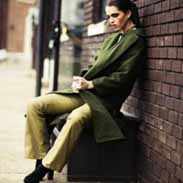# Biology Chapter 53Donna Chou
question

Population

is a group of individuals of a single species living in the same general area
question

Density

number of individuals per unit area or volume
question

Dispersion

is the pattern of spacing among individuals within the boundaries of the population
question

Mark-recapture method

to estimate the size of wildlife populations
question

Immigration

the influx of new individuals from other areas
question

Emigration

the movement of individuals out of a population and into other locations
question

Territoriality

the defense of a bounded physical space against encroachment by other individuals
question

Demography

is the study of the vital statistics of populations and how they change over time
question

Life tables

age-specific summaries of the survival pattern of a population
question

Cohort

a group of individuals of the same age, from birth until all of the individuals are dead
question

Survivorship curve

a plot of the proportion or numbers in a cohort still alive at each age
question

Reproductive table

or fertility schedule, is an age-specific summary of the reproductive rates in a population
question

Zero population growth

(ZPG), occurs when the per capita birth and death rates are equal
question

Exponential population growth

also known as geometric population growth, population increase under ideal conditions
question

Carrying capacity

symbolized by K, as the maximum population size that a particular environment can sustain
question

Logistic population growth

the per capita rate of increase approaches zero as the carrying capacity is reached
question

Life history

the traits that affect an organism’s schedule of reproduction and survival
question

Semelparity

reproduction in which an organism produces all of its offspring in a single event, also known as a big-bang reproduction
question

Iteroparity

repeated reproduction
question

K-selection

selection for traits that are sensitive to population density and are favored at high densities is known as this, or density-dependent selection
question

r-selection

selection for traits that maximize reproductive success in uncrowded environments (low densities), density-independent selection
question

Density independent

a birth rate or death rate that does not change with population density is said to be this
question

Density dependent

a death rate that rises as population density rises is said to be this
question

Population dynamics

are influenced by many factors and in turn affect other species
question

Metapopulation

when a number of local populations are linked
question

Demographic transition

the movement from high birth and death rates toward low birth and death rates, which tends to accompany industrialization and improved living conditions
question

Age structure

the relative number of individuals of each age in the population
question

Ecological footprint

summarizes the aggregate land and water area required by each person, city, or nation to produce all the resources it consumes and to absorb all the waste it generates
question

BE

Population ecologists follow the fate of same-age cohorts to A. determine a population’s carrying capacity B. determine the birth rate and death rate of each group in a population C. determine if a population is regulated by density-dependent processes D. determine the factors that regulate the size of a population E. determine if a population’s growth is cyclic
question

AE

A population’s carrying capacity A. may change as environmental conditions change B. can be accurately calculated using the logistic growth model C. generally remains constant over time D. increases as the per capita growth rate (r) decreases E. can never be exceeded
question

CE

Scientific study of the population cycles of the snowshoe hare and its predator, the lynx, has revealed that A. they prey population is controlled by predators alone B. hares and lynx are so mutually dependent that each species cannot survive without the other C. multiple biotic and abiotic factors contribute to the cycling of the hare and lynx populations D. both hare and lynx populations are regulated mainly by abiotic factors E. the hare population is r-selected and the lynx population is K-selected
question

DE

Based on current growth rates, Earth’s human population in 2012 will be closest to A. 2 million B. 3 billion C. 4 billion D. 7 billion E. 10 billion
question

EE

A recent study of ecological footprints concluded that A. Earth’s carrying capacity for humans if about 10 billion B. Earth’s carrying capacity would increase if per capita meat consumption increased C. current demand by industrialized countries for resources is much smaller than the ecological footprint of those countries D. it is not possible for technological improvements to increase Earth’s carrying capacity for humans E. the ecological footprint of the United States is large because per capita resource use is high
question

CE

The observation that members of a population are uniformly distributed suggest that A. the size of the area occupied by the population is increasing B. resources are distributed unevenly C. the members of the population are competing for access to a resource D. the members of the population are neither attracted to nor repelled by one another E. the density of the population is low
question

CE

According to the logistic growth equation dN/dt=rmaxN (K-N)/K A. the number of individuals added per unit time is greatest when N is close to zero B. the per capita growth rate (r) increases as N approaches K C. population growth is zero when N=K D. the population grows exponentially when K is small E. the birth rate (b) approaches zero as N approaches K
question

DE

Which pair of terms most accurately describes life history traits for a stable population of wolves? A. Semelparous; r-selected B. Semelparous; K-selected C. Iteroparous; r-selected D. Iteroparous; K-selected E. Iteroparous; N-selected
question

BE